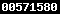### THE EFFECT OF VARIATION CONCRETE CUBE OF AXIAL LOAD ON ULTRASONIC PULSE VELOCITY TRANSMITTERhttps://doi.org/10.22146/jcef.18919

Faqih Ma’arif(1*), Priyosulistyo Priyosulistyo(2), Ashar Saputra(3)

(1) Department of Civil Engineering & Planning Education, Engineering Faculty, Yogyakarta State University
(2) Department of Civil and Environmental Engineering, Engineering Faculty, Gadjah Mada University
(3) Department of Civil and Environmental Engineering, Engineering Faculty, Gadjah Mada University
(*) Corresponding Author

#### Abstract

Nowadays, Non Destructive Tests have been applied in some quality evaluation of existing concretes on site. One of the Non Destructive Test (NDT) methods is the application of the Ultrasonic Pulse Velocity (UPV) method. In general, utilizations of UPV as one of NDT methods are to estimate the strength of concrete, to detect the presence of crack, to measure the homogeneity of concrete and the thickness of concrete plate, and also to estimate the diameter of steel attached by using UPV.
Concrete cube specimens consisted of four samples of 150x150 mm, which were control cube (KB-I), cube II (KB-II), cube III (KB-III) and cube IV (KB-IV) and were randomly taken. UPV test with a direct method was applied before and during axial load. The resultant data of pulse velocity were then calculated and analyzed by using a statistic program. The type of load applied was constant axial (0,05P0 up to 0,7P0).
The test result showed that the increase of ultrasonic pulse velocity effect on cube II due to axial load variation was optimum at 0,35P0 and was minimum at 0,7P0, if compared to the one without axial load, the results were 4,17% and 11,60 respectively. The decrease of pulse velocity on cube III due to axial load variation was at 0,25P0 and 0,7P0; if compared to the one without axial load the result were 0,47% and 20,87% respectively. And the increase of ultrasonic pulse velocity effect on cube IV due to axial load variation was optimum at 0,35P0 and was minimum at 0,7P0; if compared to the one without axial load the result were 0,52% and 21,63% respectively. The maximum limit of effective load step at structure experiencing compressive load ranged from 0,35P0 up to 0,4P0. At high stress level, the crack that occurred was spread evenly to the concrete cubic components and was giving an exponential equation y = y= 5,11e0,0467x. The result of analysis of cubes II, III and IV showed that on paired sample t-test 0,00<0,025, the significant value (2-tailed) (0,00)<(0,025); meaning there was a difference of pulse velocity due to axial load variation on concrete cube.

#### Full Text:

PDFDOI: https://doi.org/10.22146/jcef.18919

#### Article MetricsAbstract views : 907 |views : 975

### Refbacks

• There are currently no refbacks.JCEF Stats